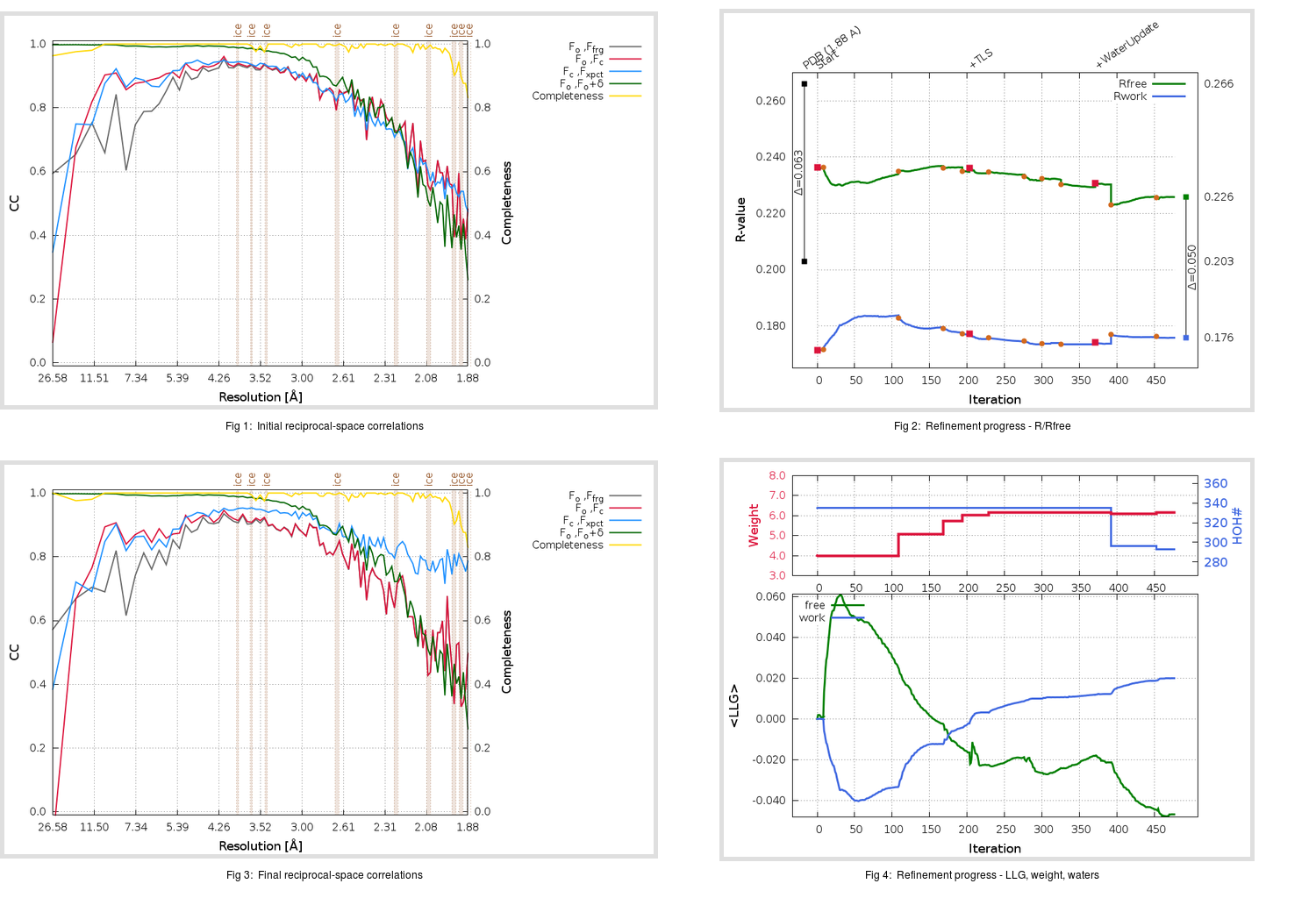Content:

## Deposited

` `
 Date deposited Date data collection Resolution R, Rfree 20200315 20200226 1.88 0.1990 0.2660

Molprobity (CCP4 7.0 version) summary:

```Ramachandran outliers =   0.33 %
favored =  95.70 %
Rotamer outliers      =   1.89 %
C-beta deviations     =     0
Clashscore            =   5.92
RMS(bonds)            =   0.0131
RMS(angles)           =   1.84
MolProbity score      =   1.83
Resolution            =   1.88
R-work                =   0.1990
R-free                =   0.2660
```

```Number of waters      =   335

<B> (all atoms) =   28.10 ( sd =    9.39 ) for       2728 non-hydrogen atoms
<B>   (protein) =   26.97 ( sd =    8.70 ) for       2370 non-hydrogen atoms
<B>     (water) =   35.67 ( sd =   10.11 ) for        335 non-hydrogen atoms
<B>    (others) =   33.17 ( sd =   14.01 ) for         23 non-hydrogen atoms

B min/max       (all non-hydrogen atoms) =   14.29 /   92.56
B min/max   (protein non-hydrogen atoms) =   15.16 /   74.92
B min/max     (water non-hydrogen atoms) =   14.29 /   92.56
B min/max     (other non-hydrogen atoms) =   22.93 /   51.69
```

## BUSTER (re-)refinement

` `

Molprobity (CCP4 7.0 version) summary:

```Ramachandran outliers =   0.33 %
favored =  96.69 %
Rotamer outliers      =   1.14 %
C-beta deviations     =     1
Clashscore            =   4.44
RMS(bonds)            =   0.0113
RMS(angles)           =   1.57
MolProbity score      =   1.47
Resolution            =   1.88
R-work                =   0.1758
R-free                =   0.2259
```

```Number of waters      =   293

<B> (all atoms) =   29.66 ( sd =    7.97 ) for       2686 non-hydrogen atoms
<B>   (protein) =   28.59 ( sd =    7.02 ) for       2370 non-hydrogen atoms
<B>     (water) =   37.75 ( sd =    9.29 ) for        293 non-hydrogen atoms
<B>    (others) =   37.24 ( sd =   16.46 ) for         23 non-hydrogen atoms

B min/max       (all non-hydrogen atoms) =   16.41 /   71.00
B min/max   (protein non-hydrogen atoms) =   17.48 /   65.01
B min/max     (water non-hydrogen atoms) =   16.41 /   71.00
B min/max     (other non-hydrogen atoms) =   23.30 /   54.41
```

Refinement progression:Results:

` `
 File Remark 5RE4_aB_refine.01_03_refine.pdb.gz exact refinement commands are in header 5RE4_aB_refine.01_03_refine.mtz.gz including original deposited data and several re-refinement map coefficients 5RE4_aB_refine.01_03_BUSTER_model.cif.gz including any non-standard compound restraints 5RE4_aB_refine.01_03_BUSTER_refln.cif.gz